November 30, 2006

SCRIBE POST NOVEMBER 17

Hey everyone its Gerald. I understand my scribe post is pretty late and i'm very sorry.
To get things started Mr. K put some questions on the board for us to do.

HOW MANY WAYS CAN 5 PEOPLE (A,B,C,D,E) SIT IN A ROW IF A MUST:

A) SIT NEXT TO B
B) NOT SIT NEXT TO B
C) SIT TO THE LEFT OF B BUT NOT NECESSARILY NEXT TO B

SOLUTION:

A) 4!2! = 48 ways to be seated in a row if A must be seated next to B

2! = AB seated next to eachother
4! = C,D,E,AB = since AB must be seated together

B) 5! = 120

120 - 48 = 72 ways to be seated not next to B

5! = all ways they can be seated regardless who they sit with
48 = the ways AB are seated together

C) 5! divided by 2 = 60 ways A sit next to be but not necessarily next to B

5! = all ways they can be seated regardless who they sit with
2 = A sitted to the left of B

IN HOW MANY WAYS CAN 5 PEOPLE SIT AT A ROUND TABLE

the formula for this is (n-1)!

(5-1)! = 4!
4! = 24 ways 5 people can sit around a table

MATH DICTIONARY NOTES:

CIRCULAR PERMUTATIONS

the number of ordered arrangements that can be made of "n" objects in a circle is given by:

(n-1)!

example: how many ways can 6 people be arranged around a circular table?
(6-1)! = 5!
5! = 120 ways 6 people can be arranged around a circular table

SPECIAL CASE: a bracelet is a circle that can be flipped over. The number of arrangements that can be made of object on a bracelet is:

(n-1)! divided by 2

example: how many different bracelets can be made using 5 different coloured beads?
(5-1)! divided by 2 = 4! divded 2
24 divided by 2 = 12 ways a bracelet is made using 5 beads

thanks and sorry once again. I hope everyone did good on the recent test we just had. See everyone next class. Good night and goodbye =)

scribe post for today

Another winter day had past and we had an uneasy day due to the test we had this afternoon. In our first period pre-calculus, we had a pretest in relation to our actual examination where we did individual worksheets then group work and then a two and a half minute spy time. After that Mr. K revealed the answers for the following questions:

1) 7 teams compete in a men's hockey league. If each team plays each other twice, how many games are necessary to complete the league's schedule?

(a) 42
(b) 21
(c) 84
(d) 52

The answer is (a) 42 because the solution is "2*(7C2)" where 7 is the number of teams and there must be at least first two teams to compete then multiplied by 2 because they must compete with each other twice.

2) The sum of the seventh row of Pascal's triangle is the same solution to:

(a) The number of solutions a student could get if they guessed on six questions of a multiple choice exam, each question having four answers.
(b) The number of solutions a student could get if they guessed on six questions of a true false exam.
(c) The number of solutions a student could get if they guessed on seven questions of a multiple choice exam, each question having four answers.
(d)
The number of solutions a student could get if they guessed on seven questions of a true false exam.

The answer is (b) because in six questions there are two choices for each, which is either true or false. Therefore, 2*2*2*2*2*2 or 2^6 equals 264.

Solution:
_ _ _ _ _ _
2 2 2 2 2 2 = 264

3) Suppose the last four digits of a telephone number must include at least one repeated digit. How many such numbers are there?

The answer is 4,960 because there are 10^4 or 10,000 ways of having last four digit numbers if there is no restriction and there are 10*9*8*7 or 5040 ways of having last four digit numbers with restriction. In order to solve for the number of ways to have last four numbers with at least one repeated number, we have to subtract 5,040 from 10,000. As a result, we got 4,960.

Solution: 10,000-5,040= 4,960

4)A multiple choice exam has 20 questions, each with four possible answers, and 10 additional questions, each with five possible answers. How many different answer sheets are possible?

There are (4^20)*(5^10) or 1.073741824 x 10^19 possible answer sheets because in 20 questions there are four possible answers, 4^20, for each and in 10 questions there are five possible answers, 5^10, for each. In order to get the answer we have to multiply both (4^20) and (5^10) with each other because we are looking for the differenr number of answer sheet possible.

Solution:
_ _ _ _ _ _ _ _ _ _ _ _ _ _ _ _ _ _ _ _
4 4 4 4 4 4 4 4 4 4 4 4 4 4 4 4 4 4 4 4= 4^20

_ _ _ _ _ _ _ _ _ _
5 5 5 5 5 5 5 5 5 5= 5^10

(4^20)*(5^10)= 1.073741824 x 10^19

5) In a 52 card deck, how many 5 card poker hands that have exactly two pair? one pair?

in two pairs: There are 123,552 possible cards with exactly two pairs.

Solution: 13C2* 4C2* 4C2* 11C1* 4C1= 123,552

where:
13 is the number of face values from ace to king
4 is the number of suits per face value (hearts, clubs, diamonds, and spades)

13C2 is the equation for a person to choose 2 face values out of 13. Two 4C2 are given because when a person have already chosen two face values, he will then choose one pair of number from four suits, which are the hearts, diamonds, clubs, and spades and another pair from the other suit. 11C1 is the equation for the remaining face values where the person has to choose only one from it. 4C1 is given because after the selection from the remaining 11 face values, the person has to choose one number from any suits of the same number.

in one pair: There are 1,098,240 possible cards with exactly one pair.

Solution: 13C1* 4C2* 12C3* 4C1* 4C1* 4C1 or 13C1* 4C2* 12C3* (4C1)^3= 1,098,240

13C1 is the equation for the 13 face values where a person has to choose only one from it. 4C2 is given because after he has chosen the face value, he has to choose one pair from four different suits of the same number. 12C3 is the equation for the remaining face values where a person has to choose three from it. Three 4C1 are given because in different face values there are four suits each and he has to choose only one number from the four suits of the same number resulting into having three different numbers.

In our second period pre-calculus, we started answering our Combinatorics test where all of us are hoping for the best test results.

That's all for now guys.
The next scribe is Allen.
Goodluck to all of us.....
God bless you all..........

------(",) daphne.......

Jho's Bob =)

Well this unit was an okay unit for me, it was kind of a refreshment of what i left of last year. I remember last year this was one of my best units, but for this year, i've learned some new stuff, like the pascals triangle and the bionomial expansion, unless i just forgot. But yeah i think i've learned alot more this year about this unit when i was teached about it last year. I hope i pass the test, i'm not oh to convinced that ill be superior for this quiz, because of my absenses and my failure to keep up with what we learnt. But i will try my best.

Oh yeah sorry about not posting up a scribe post yesterday, i couldn't get my internet going, after work, but i have informed who will be the scribe for today. I'll try to have my scribe up ASAP. GOOD luck everybody

JessicaJill's Blogging on Blogging

So, the test is tomorrow, and the question is, am I prepared? Well, despite my being absent from school for over a week, I feel comfortable with this unit. It does get a little tricky every now and then, but I think that just a little more effort will help me through. I'm just hoping I do as well tomorrow as I did on my quiz tonight. Last year I think I had some trouble with this unit, probably because of my excessive absenteeism, and I'm not being cocky, but I feel like I'll do exceptionally well with our test.

Good luck everyone.

November 29, 2006

Ashley's BOB#4

Okay so this unit is alright. I mean I liked it at first when we started learning it because it seemed simple but now i'm not so sure. after doing the quiz i'm not sure how well i'll do on the test. i guess i still get confused on the pick and choose stuff. like i'm not sure which one to choose even though i know that you choose, choose when order doesn't matter and pick for when order does matter but then i start double guessing myself on deciding whether the question says that order matters or not. i don't know anymore but i still like this unit for some reason even though my brain hurts everytime i'm in class=/ oh well....just means i have to practice more on the questions. Good luck guys!

azn_chilly's blog'n on blog'n

this unit was somewhat difficult and confusing on some parts, like on choosing on which formulas to use for which questions. but i get most of it but then suddenly i think its a different answer and all of them are tricky at the start but i read more carefully the understanding for it gets to me then i can be able to answer it, but practice and practice make better then say and its working for me for some questions......

thats all i think i have to say at this point so...

goodbye to all & good luck to all on the pre-test and real test hahhah uhhhhh so...
latez to allBlogging on Bloggnig

I did about average for this particular work, but then again I've never been very good at making estimates about myself. Homework I went through to try and understand as much as possible and at the least the only thing I think I have to worry about is where to use the pick formula and where to use the choose formula. Truthfully I kinda wish we had some more time before the test but that's how I feel about all my tests.

jessica.'s blogging on blogging.

perms and combs.. what a great unit. i actually understand this more than last year. this year we went through this unit more throughly. there are some problems that i would get.. like when to use the "pick" or "choose", sometimes you just have to re-read the question a couple of times to get it. i think that's all that's bothering me at the moment =/ goodluck on the test tomorrow (:

gooday&goodnight.

blogging on blogging

at first, I'm having fun in the lesson. but when the time past, i getting confused in what we are discussing. it really gave me a hard to understand it because i can say im a slow learner. when i i'm doing my homework. its a great help for me. before the unit end. I understand everything then. Its just a matter of practice.
good luck 2 everyone. enjoy your day.
Hope I'll pass this test.

Richard's BOB on combinatorics

This unit, Combinatorics, is an interesting unit. It doesn't give me stress at all. But be careful...because it is tricky. You don't need to follow the formula. Just carefully analyze the problem and you will surely get the correct answer. I'm having trouble about arranging things with different kind of restrictions. I'm always got stuck on how to deal with those problems. Pick and choose is easy as long as you know which one is to use.
In this unit, I also learned about patterns especially the Pascal's triangle. Binomial Expansion theorem is also a great lesson. In card problems, I'm familiar with the terms that is being used. But it seems like it is still complicated.

Good luck everyone for the test! I hope we all did not just good but the BEST!

BLOGGING ON BLOGGING

Holy moly ! it's not even funny, this unit went by fast, but I mean, I didn't get much, the beggining made a it of sense, then I lsot myself somewhere, tried to do some homework and I got what was happening, but then once we got in class, I totally dunno what was going on, wowsers, I need to study hardcore tonight! but good luck to everyone else ! =).

bob

This unit was pretty straight and went by really fast...the only problem I have with this unit is when to use "pick" and "choose" because for the bus problem we used "pick" so does that mean we use "pick" for the desk questions too? because what if the student wanted to sit in a particular desk, how are we to know, and for the bus question how do we know if they want a specific seat like one near the window?...and for the quiz I thought I seriously knew all the answer but it didn't turn out that way, which surprised me. As for when we draw the "slots", how do we know when to do that or factorial...because on the quiz I did a question in factorial and the answer didn't turn out right. Anyway I'm sure it'll be fine because I understand all of the group work and in-class board questions..well good luck to everyone and as Mr.K says it "may the best news be yours" =)

BOB time again!!!

I would just like to say that this has definitely been my favorite unit!! I have also done permutations and combinations before, but alas my teacher didn't exactly explain it.. at all... which meant it was really hard for me to understand... luckily mr. k is sooo much better at making things easier to understand!!! this unit actually made total sense to me and instead of feeling like i was completely lost all the time, i actually answered some questions!! woo hoo... and i've already done much better on the online quiz then i did during logs so i feel much better... with a little more practice on my part, and some discussion in class first class tomorrow, i think i might actually do well on the unit test... which would be nice for once... i really need to get my mark up, and i'm going to have to work on it, but i think i can do it if i really try!! ok well i'm done, yay for permutations and combinations... i hope i didn't just jinx myself for the test :P ... ah ha.. well good luck everyone and i'll see you in the morning!!!

BoB- counting(combinatorics)

well I can definitely say that i understand counting a whole lot more than last year. but I'm still having trouble on problems that involving playing cards. like what you need to you need to think about first IE, suits, face values etc. the binomial theorem is easy up until you have to find the term containing something like x^20 given (x-y^4)^6. i know how to find the middle term now which is uber easy. I think that's pretty much it i can't really grasp the concept of how to deal with playing card problems.

Counting 1..2..3!

At the beginning of today's class, the class was divided into 5 groups of 4. Each group were given the same 5 questions on counting. As seen below. I will now explain briefly how to get these answers and why.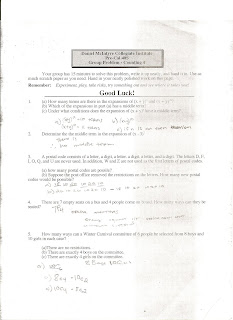Solutions and Explanations

1.a) As seen in question one the answer is 21 terms. Determining how many terms there are is defined by (n +1) , n is the exponent after the binomial expansion. So ( 9+1) =10 and (10 +1)=11. Therefore 10+11 = 21 terms.

1.b) The answer is (x +y) ^10. This is because in order for you to have a middle term, you need to have the same amount of numbers before and after that middle number, and this can only be done with an odd number of terms, but since our formula is (n+1), in finding how many terms, in a binomial expansion it would have to be an even number. (x +y) ^10, has 11 terms therefore having 5 before and 5 after. The middle term would be 6 in this expansion.

c) As said above, If "n" is an even number.

2. The answer is there is no middle term because the "n" was an odd number.

3. a) self explanatory. (18)(10)(20)(10)(20)(10) <given to us,and 10 stands for numbers you can choose between 0-9.

b) KEY WORD IS NEW. So without restrictions it would look like this (26)(10)(26)(10)(26)10) but since how many new ones are formed you would subtract the answer from, part a, from the "no restrictions" to get all the new codes.

4. It is 7P4. It is pick, because order matters, enemies do not want to sit beside enemies, or window sitters. 7 stands for the empty seats the can pick from, and 4 stands for the people who will be sitting in their picked spots.

5. a) 18C6 because order does not matter. 18 stands for number of people. 6 stands for people choosen to be on committee.

b) 8C4(10C2), exactly 4 boys, so since there are 8 boys they have to choose exactly four, and remaining 2 people will have to be chosen from the 10 girls, to make the committee of 6 people.

c) 10C4(8C2)Same explanation as above, but exactly 4 girls have to be chosen from the 10 girls , then the other 2 people will have to be chosen from the 8 boys, to form the committee.

Counting Quiz

As we discussed in class today you will be writing an online quiz in preparation for the test tomorrow. Here's what you have to do to get started:

Write the quiz online here. It is live now and will no longer be available as of midnight on tonight. When you get to the site follow these instructions:

If you hit any snags email me and we'll sort it out together.

This quiz is timed. You'll only have 45 minutes to complete it once you've begun. It consists of 13 multiple choice questions. DON'T PANIC and I know you'll all do well. We'll talk more about this in class tomorrow. ;-)

Remember ...

There are three kinds of people ... those who can count and those who can't. ;-)

November 28, 2006

Counting Problems

Okay, today Mr.K explained more about the online assignment we had to do on the Flickr site. For anyone who wasn't in class, you have to get one picture that represents something of Trigonometry. It has to be in the real world, not something drawn up and then photographed, but you can also set it up how you want it to be. Just remember it has to be a trigonometry picture; it could represent a triangle, a sine graph, angles, area, degrees, etc. When you get your picture try to incorporate as many different concepts of trigonometry as you can. After showing us he went to putting up the questions on the board.

1. From a 52 card deck, how many 5 card hands have:

a) 3 hearts and 2 clubs?

b) 4 of a kind (4 cards, same face value, and 1 card of a different face value)

c) Royal flush (A, K, Q, J, 10, with the same suit)

d) straight flush (5 cards, in sequence, of the same suit)

2) Find the 6th term of (x - 2y)^9

3) Find the middle term of (3a + 2b^2)^6

4) Which term of (x^2 - 1/x)^6 is constant?

1. a) 13C2 . 13C3 = 22 308

b) First we got the total number of hands dealt
52C5 = 2 598 960
13C1
We get this value because of the 13 different face values
4C4
We get this value because there are four suits in each face value, and since we need four ofa kind it's 4C4.
12C1
Since this choice is supposed to be a different suit then one of the face values will be eliminated
4C1
We only need one of the face values and only one suit.

Afterwards we just multiply it all together:

13C1 . 4C4 . 12C1 . 4C1 = 624

1 . 4c1
There reason we are multiplying 4c1 by 1, is because there is only one way to get the sequence
4C1 just means that it could be any one of the 4 suits.

d) This one is different because you don't count the royal flush.
This problem is also related to a problem we did in previous classes, where there were 4 students and 9 seats to fill.
9 . 4C1
Because there are nine different ways to get the sequence, and 4C1 means that the whole had has to be one suit.

2) To find the sixth term of (x - 2y)^9, you have to remember that 9C0 also counts as a term.
Solution:
9C5 (x)^4 (-2y)^5 = 126 x^4 (-32y^5)
= -4032 x^4 y^5

3) Let x = 3a y = 2b^2
6C3 x^3 y^3 = 20 (3a)^3 (2b^2)^3
= 20 (27a^3) (8b^6)

4) 1st term:
6C0 (x^2)^6 = x^12
2nd term:
6C1 (x^2)^5 (-x^-1)^1 = x^9
3rd term:
6C2 (x^2)^4 (-x^-1)^2 = x^6
...
The 5th term is the constant term.
6C4 (x^2)^2 (-x^-1)^4
= 15 <--- This is the value for the constant term
(a + b)^p
pCd (a^c) (b^d)
c + d = 6
2c - d = 0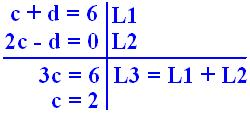SECOND CLASS...

1. (2x^3 - 1/x^2)^10 Find the constant term

2. The 4th term of (x - 1/2)^n is -15x^7, find n.

3. How many ways can a person get from point A to B?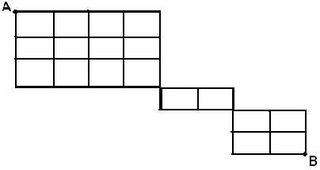4. In a 52 card deck, find the number of ways to get a 5 card hand that is:

a) a full house (3 cards of one face value, 2 of another)

b) Flush (5 cards, same suit, not in sequence)

c) Straight (5 cards in sequence, not all the same suit. Ace high or low)

d) 3 of a kind

e) Two pair

f) One pair

g) No pairs

10C6 a^4 b^6

10!/(6!4!) (2x^3)^4 (-x^-2)^6

210 (16x^12) (-x^-12)

3360

An extra question...Find the term that contains x^15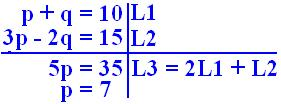10C7 (2x^3)^7 (-x^-2)^3

-120 (128x^21) (-x^-6)

-15 360 x^15

2. nC3 a^(n-3) b^3 - - 15x^7

n!/((n-3)!3!) (x)^(n-3) (-1/2)^3

x^(n-3) = x^7

n-3 = 7

n= 10

7!/(4!3!) = 35

Going right, then down.

We get 4! because you're going RRRR. You're making four rights on the squares.

3! because you're going DDD. You're down 3 squares.

The combination would be RRRRDDD, it's how you're getting from point A to B.

Another way was using Pascal's Triangle: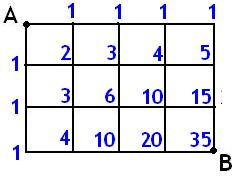As you can see, when you look at it at an angle, you can see pascal's triangle.

B) RD = 2

c) RRRRD----5!/4 = 5

d) RRRRDD . RRD . RRDD

6!/(4!2!) . 3!/2! . 4!/(2!2!)

15 . 3 . 6 = 270

4a) 13P2 . 4C3 . 4C2

We used 13P2 because the order does matter.

b) 4C1 . 13C5 - 10.4C2

the 10.4C1 is the total number of flushes in sequence.

Well that's the end of my scribe. I hope it was informative to all of you. The next scribe shall be......Jhoann.

November 27, 2006

Can you say..... Patterns are IMPORTANT??Hi guys its me again!! I hope everyone is enjoying their evening... so
this is what we did today in class.....

We started class off by going over some of the things we did last
Thursday, so Mr. K wrote these on the board.

(a+b)^0 = 1
(a+b)^1 = 1a+1b
(a+b)^2 = 1a^2+ 2ab+1b^2
(a+b)^3 = 1a^3+ 3a^2b+ 3ab^2+ 1b^3
(a+b)^4 = 1a^4+ 4a^3b+6a^2b^2+4ab^3+1b^4

1
11 1
11211
1 13 131 1
11 4 16 14 11
1115110 10 15 11

oCo
Co 1C1
2Co 2C1 2C2
3Co 3C1 3C2 3C3
4Co 4C1 4C2 4C3 4C4
5Co 5C1 5C2 5C3 5C4 5C5

Do you remember those... if you don't you might want to
check Ashley's lovely scribe post from yesterday for reference

After he finished putting all of these back up on the board, we went
over patterns that we fount yesterday like:

Powers of 2
1
1 1
1 2 1
1 3 3 1
1 4 6 4 1
1 5 10 10 5 1
2^3 = 8 111111111111+3+3+1= 8
2^4 = 16111111111+4+6+4+1= 16

And powers of 11

1
1 1
1 2 1
1 3 3 1
1 4 6 4 1
1 5 10 10 5 1

11^2 = 121
11^3 = 1331
11^5 = 161051

Whoa that doesn't look

the same... well it still works, you just
have to make sure to do the carrying.
The 5 turns into 6 and the tens turn
into 0 and 1 because 10 carry the 1
leave the 0, and 11 carry the 1 and
leave 1 behind and that 5+ the carried
1 = 6

Ok now here's the new pattern that Mr. K showed us... it shows the
Fibonicci numbers in Pascal's triangle (even though it wasn't pascal

Pay attention cause its hard to see at first!!

The fibonicci numbers can be found on the diagonal edges... the numbers that are cut by this line are added together to show these fibonicci numbers.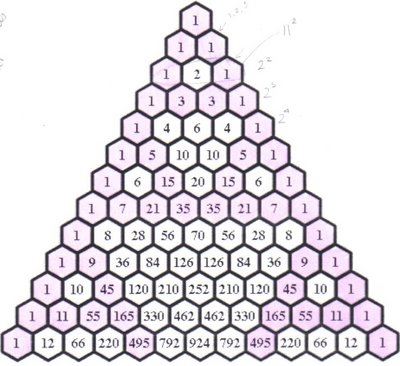Can you see the diagonal edges... they are the pink lines.... the first line crosses through only 1... the next crosses only 1... the next passes through 1 and 1... add these together to get 2... the next row passes through 1 and 2.. add these together to get the 4th fibonicci number 3... and on and on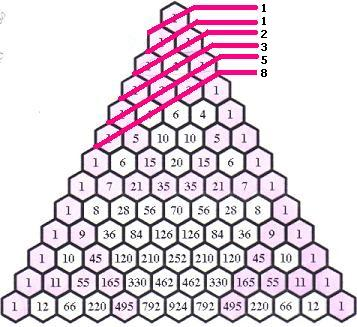Can you see it?? if not feel free to come and ask me in class, i'll do
my best to show you and make sure you understand.

After everyone found this pattern Mr. K wanted to point out other interesting
facts... so he told us to take out our calculators...

if you take one fibonicci number, and divide it by the fibonicci number before
it, you will find that as you travel down the triangle, the values get closer and
closer to the "GOLDEN RATIO" or PHI !!! ∅(phi) = 1.61803399

1/1 = 1
2/1= 2 Can you see how the numbers seem to be
3/2= 1.5 converging (narrowing down) to a single value?
5/3= 1.6667 All getting closer and closer to
∅!!
8/5= 1.6
13/8=1.625

As I've already mentioned, ∅ is the GOLDEN RATIO... but why is
this so you ask... well because if an item has a ratio that is equal to, or
close to
∅ than it just looks better... unexplainably but it really does..

For example have you ever wondered why 4 by 6 pictures look better
than 5 by 7s... they're smaller, yet they look better... this is because

6/4 = 1.5 * because this has a ratio closer to ∅ it is more visually appealing*
7/5 = 1.4

Another example of this is the Parthenon. This is a temple in Greece dedicated
to the goddess Athena (who is the goddess of wisdom). This temple is also
known as 1 of the 7 wonders of the world as it is one of the most visually
appealing structures in the world. This is because if you look at the Parthenon
it is constructed of rectangles. If you take the larger length of each rectangle and
divide it by the shorter length you will find that the ratio is 1.618... or really
close to the GOLDEN RATIO !

Everything is connected!!! The Fibonicci ratio is not only encoded Pascal's
Triangle, but it is also in various aspects of life, including the human body.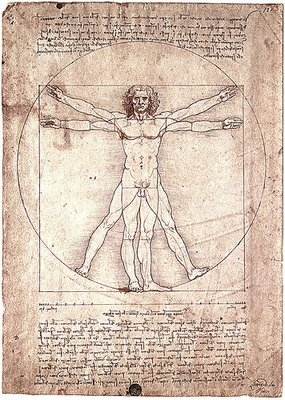This is the Vitruvian Man done by Leonardo Davinci. (Found on Flickr,
posted by absolutesteven) It is known as the most accurate drawing of
a human being ever done. Why is this... i bet you can guess... that's right
Davinci made sure to measure every and make sure that every
part came out close or equal to the GOLDEN RATIO ....

ex.
Man's height / distance from ground to navel
Man's arm / distance from elbow to tip of middle finger

Don't you just love it when everything fits together like that?? :D

After his speech on the GOLDEN RATIO, Mr. K brought us back to
the (a+b)^x expansions....

What are the patterns???

1
1 1
1 2 1
1 3 3 1
1 4 6 4 1
1 5 10 10 5 1

oCo
1Co 1C1
2Co 2C1 2C2
3Co 3C1 3C2 3C3
4Co 4C1 4C2 4C3 4C4
5Co 5C1 5C2 5C3 5C4 5C5

Firstly the co-efficents for the expansion can be found within
the
triangle (look at above triangle with matching colour)

(a+b)^4 = 1a^4+ 4a^3b+6a^2b^2+4ab^3+1b^4

Can you see the co-efficents??
1, 4, 6, 4, and 1
according to the 5th row

and 4Co row...
tells us the exponents for the expansion

a^(e-x) b^x
e = what it is being chosen out of.. in this case 4

4Co 4C1 4C2 4C3 4C4

so this expansion is

a^(4-0) + a^(4-1) b^1 + a^(4-2) b^ 2 + a^(4-3) b^3 + a^(4-4) b^4

a^4 + a^3b + a^2 b^2 + a b^3 + b^4

If you add these two patterns together you will get...

1a^4 + 4 a^3b + 6a^2 b^2 +4 a b^3 + 1b^4

the expansion of (a+b)^4

Now, if asked to find the 3rd term of (a+b)^4 do you have to
figure, and write out the whole expansion. Sure why not,

(a+b)^4 is easy... but what if i asked for the 7th term of
(a+b)^11?? do you really want to write that out??

Ok now its time to show you how to do this much QUICKER
and much EASIER....

First of all i must make sure that it is clear to you that as we go along
the triangle from the left to right the terms are numbered from 1
increasing... 1, 2, 3, 4, 5 etc.

oCo
1Co 1C1
2Co 2C1 2C2
3Co 3C1 3C2 3C3
4Co 4C1 4C2 4C3 4C4
5Co 5C1 5C2 5C3 5C4 5C5
11112222333444455556666
So,
5Co is term 1
5C1 is term 2
5C2 is term 3
5C5 is term 6

Do you see the pattern, the term number is always 1 larger
than the amount being chosen!!

Therefore, if the number being chosen is always 1 larger,
when we ask for the Xth term, we must subtract 1 so it will
look like this nC(x-1) where n equals the amount being
chosen from.

Ok given this question, 7th term of (a+b)^11
lets use what we know, and the new equation

11 is the number being chosen out of and takes the place of
the n. looking like this 11C(x-1)

7 is the term we want, and takes the place of x.
looking like this 11C (7-1)

Do the math... 11C6 is what you are left with. Now use
rules from before that state that give exponents to a and b

a^(e-x) b^x
e = what it is being chosen out of.. in this case 11
b = the number chosen, in this case 6
a^(11-6) b^6

a^5 b^6

Now put both portions together!!

11C6 a^5 b^6
This will be the 7th term in the expansion of (a+b)^11

Lets try this again to make sure you've got
it....

find the 5th term of (a+b)^13

13 is amount being chosen from
5-1 = 4 this becomes the number being chosen!!
Therefore this becomes 13C4

next comes the exponents
13-4 = 9 which becomes the exponent on a
4 is the exponent on b

put them together

13C4 a^9 b^4

is the 5th term of (a+b)^13

Mr. K tested the class a few more times, and soon a couple
people could find the Xth term of an expansion in less than
10 seconds, and the rest of the class could do it in under
30 seconds.... can you?? Time yourself... If you work at
it you'll get there... if you have any questions just ask me
i'm always willing to help if i can!!

Ok guys time to turn up the heat!!

How many terms are in each expansion?? How do we know this??

Well the number of terms in an expansion can be found by taking the
power of the expansion...

ex. (a+b) ^11
the power is 11

take this power and add 1
11+1 = 12

12 is the number of terms in the expansion!!

What would happen if we were asked to find the middle term of the
expansion??

for odd numbers this is easy, all you need to do is add 1 to the power,
then divide by 2

13 +1 = 14 14/2 = 7

Therefore the middle term in this expansion is the 7th term!!

* this formula will only work if the power you start off with is odd*

In order to find the middle term for an even power, we take the
power, divide by 2 and add 1

12 / 2 = 6 6+ 1 = 7

Therefore the middle term in this expansion is the 7th term!!

* this formula will only work if the power you start off with is even*

Now that we can find the middle term of the expansions we've practiced,
can we find the middle terms of other binomial expansions??? YES!!!

how about [x-(2/x)]^7 ?? what is the middle term of this??

First of all lets make this into something we can understand shall we??

Let a = x
Let b = - 2/x to make this even simpler we're going to change
this into - 2x^(-1)

so now the binomial looks like this

(a+b)^7 and that is much better....

now find the middle term... 7 is odd therefore we must add 1 to make 8 and divide by 2.. the middle term is 4!!

now show the term 4 of this expansion.

7C3 a^4 b^3 *reference above if you don't remember how
1111111111111to do this step*

now rewrite 7C3 in terms of factorials....

7! / (4!3!) a^4 b^3
4! reduces leaving--> 7x6x5 / 3! a^4 b^3
6 and 3! reduce as 6 = 3! leaving--> 7x5 a^4 b^3
35 a^4 b^3

Now substitute the real values of a and b back in.

35(x)^4 (-2x^-1)^3
35 x^4 (-8x ^-3)
-280x^1

therefore -280x^1 is the middle term in the binomial expansion
of
[x-(2/x)]^7

Lets try this again to make sure you've got it!

Find the middle term of (x^2 - 2/x)^8

now make this simpler for yourself
Let a = x^2
Let b = -2/x even simpler -2x^-1

now the binomial looks like

(a+b)^8

middle term for the power of 8 is the 5th term
therefore 8C4 a^4 b^4

write in terms of factorials

8! / (4!4!) a^4 b^4
4! reduces leaving--> 8x7x6x5 / 4! a^4 b^4

Now substitute the real values back in

70 (x^2)^4 (-2x^-1)^4
70 x^8 (16x ^-4) 111
1120x^4 1111111111
1111111111111111

A common mistake made is when the exponent
is an even number, the student forgets to make
the coefficient positive. Watch for this!!

Therefore 1120x^4 is the middle term in the binomial
expansion of
(x^2 - 2/x)^8

CONNECTIONS ARE EVERYWHERE!!!!!!

As you have seen through class and scribe posts for this
unit, everything is connected and therefore

Combinations and binomials go hand in hand!!

The last of the class was spent reviewing most of the above one more time, in order to really make sure that we understood!!

I hope everything in this scribe post is useful to you. If you have any questions or concerns don't hesitate to ask me, I'm here to make this scribe post make perfect sense to you!! Enjoy the rest of your evening, and i'll see you all tomorrow!! Good Night!!

p.s please make sure to have at least 5 pictures on your flickr account if you are a new user. If you do not, your account will not be reviewed, and therefore not deemed safe to make your pictures public for the assignment!!

Oh ya... and just to finish this off, jessiccal_198 your scribe tomorrow :)

Ok i believe that the spacing is fixed now... see you later!!

November 26, 2006

Hey it's Ashley and I'm the scribe for today and yes I know i didn't do it right away but for those who missed you should have really been there. It was a pretty interesting class because the class was all about PATTERNS and mr.k telling us about how God built the universe and blowing our minds (not literally of course...)

To start off Mr.k posted up the questions that he previously posted the day before on the board.

Expand and Simplify

(a+b)^0
(a+b)^1
(a+b)^2
(a+b)^3
(a+b)^4

To answer this question it's simple just expand and simplify

(a+b)^0 = 1 (because its exponent is 0)
(a+b)^1 = a+b (because its exponent is 1)
(a+b)^2 = (a+b)(a+b) => a^2 + 2ab + b^2

now be smart about the next question
instead of doing this (a+b)(a+b)(a+b) just multiply a^2 + 2ab + b^2 by (a+b) since it will be easier since a^2 + 2ab + b^2 is the same as (a+b)(a+b) and you are just multiplying one more (a+b) since its to the power of 3.
(a+b)^3 = a^2 + 2ab + b^2 * (a+b)
now distribute the a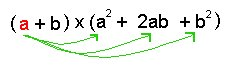= a^3 + 2a^2b + ab^2

then distribute the b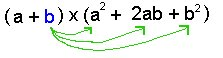= a^3 + 2a^2b + ab^2 + a^2b + 2ab^2 + b^3

then gather the like terms
= a^3 + 2a^2b + ab^2 + a^2b + 2ab^2 + b^3
= a^3 + 3a^2b + 3ab^2 + b^3

Now do the same for (a+b)^4. Distribute and gather the like terms.
*Do not foil because foiling only works with binomials*

(a+b)^4 = (a^3 + 3a^2b + 3ab^2 + b^3) * (a+b)

Distribute a
= a^4 + 3a^3b + 3a^2b^2 + ab^3

Then Distribute b
= a^4 + 3a^3b + 3a^2b^2 + ab^3 +a^3b + 3a^2b^2 + 3ab^3 + b^4

Gather the like terms
= a^4 + 3a^3b + 3a^2b^2 + ab^3 +a^3b + 3a^2b^2 + 3ab^3 + b^4
= a^4 + 4a^3b + 6a^2b^2 + 4ab^3 + b^4

The other question posted on the board
Look for a pattern

Write the next two rows

1
1 1
1 2 1
1 3 3 1
1 4 6 4 1

Now looking at this question having down the previous question a few of us realized the pattern

The first pattern:

Adding the numbers like this (sorry it's hard for me to explain so a diagram will hopefully help):

The Second Pattern:

The numbers in the triangle are the coefficients to the expand and simplify question.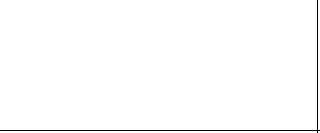The Third Pattern:

The exponents on the variable are: a descending and b ascending.
example: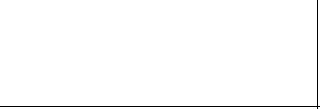The last question posted on the board:

Evaluate each term (you can use your calculator):

OCO
1CO 1C1
2CO 2C1 2C2
3CO 3C1 3C2 3C3
4CO 4C1 4C2 4C3 4C4

Now if you did this on your calculator you would get

OCO = 1
1 = 1CO 1C1 = 1
1 = 2CO 2C1 = 2 2C2 = 1
1 = 3CO 3C1 = 3 3C2 = 3 3C3 = 1
1 = 4CO 4C1 = 4 4C2 = 6 4C3 = 4 4C4 = 4

In other words.....

1

1 1

1 2 1

1 3 3 1

1 4 6 4 1

It's the same as the second question. Neat huh?!

It's interesting as to how all of the three questions written on the board are all related to each other somehow. So how is the last question connected to the first question? Well.... it's better if i show an example: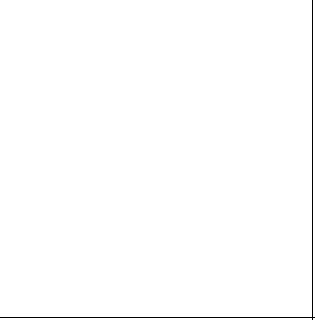Then just when I thought there couldn't be more to talk about Mr. K handed u
s a worksheet which I'll post tomorrow. But it basically showed Pascal's Triangle which is this and I forgot what Mr.k said about pascal so ask him if you want to know who he is.Now at the bottom of the worksheet it asked if you can find powers of 2, powers of 11 etc....

To find the powers of 2 in the triangle you just add the numbers in each row.To find the powers of 11 in the triangle just look at it.Sierpinski triangle is a fractal and well...... a guy named Sierpinski decided to punch out triangles in an equilateral triangle where the area is fixed but the perimeter is infinite. I think that's what Mr.k said but here's what Sierpinski did. He used an equilateral triangle and punched out other equilateral triangles inside it like this: (sorry bad explanation)Then Mr.K told us to shade in the odd numbers on the triangle which felt like I was back in elementary. We should color more often!Looks like the Sierpinski triangle doesn't it? If you continued on shading the odd numbers it would be even more clearer. Cool right?!

Next was on the talk about the Fibonacci numbers 1, 1, 2, 3, 5, 8,....

Fibonacci numbers work by adding the last two previous numbers.

1+ 0 = 1, 1+1 = 2, 1+2 = 3, 2+3 = 5, 3+5 = 8

Apparently bees, yes bees follow the fibinacci sequence because a male has one parent which is it's mother and a female has two parents, both a mother and a father.

What else was mentioned in class? Ah yes flowers also respect the fibonacci sequence. You won't find a flower with 6 petals but you will find a flower petal with 5 petals but you won't find a flower petal with 7 petals but you will find a flower with 8 petals, so that's why it's rare to find a four leaf clover.

And Lastly Mr.K mentioned a number which i forgot what the number is called but it has to do with something about dividing your body into parts and it always equals to that number. Sorry but i forgot what he said but it sounded cool. However I'm still not sure as to what part of the lesson was on God building the universe? Oh well....

The next scribe will be.........LISA!!!

and sorry about the picture not showing. i wonder why that is?

November 22, 2006

Countings and Combinations

Hi im John,the scribe for today.
In today's class, we started our lesson about a chance of winning 6/49 lotteries.
Mr. K explain to us our chance to win in the lottery. i'll give you some of his explanation.
In 6/49, we have to choose 6 numbers in order for us to get the ticket.
Here is the explanation in the problem.
Assuming there's 49 letters word, 6 of that is the one we like and the rest are those we doesn't chose..

49 C 6 = 49! / (49-6)! 6!
= 49! / 43!*6!
= 13,983,816 no. of ways tickets can be sold in lotto 6/49.
So, if you buy 1 ticket: 1 / 13,983,816 is your chance.
: 7.15 x 10^-8
: 0.0000000715 % a chance in choosing a number in lottery.

He ask the class, do you still buy a lottery? For me, Yes. I will. because still i have a single chance to win in the lottery and maybe that's the time what I'm waiting for.
Anyway, back into class..
If we have 20 people, can we still do to become a lottery winner assuming the pot money is 14 million or above.?
let say, every 15 sec. is the time of processing the ticket.
so 15*4 is 60.this is minutes.
60mins = 1 hour therefore 24x60 = 1440 tickets can be print in an hour.
1440 x 24 = 34560 because we're looking for day.
the answer multiply by 20 people equals 691200.
therefore:
13983816 / 691200 = 20.23121528
We say that there are 20 days will take to get the lottery card to finished.

Again, the question is, are u still going to buy a ticket because there's just 4 days to wait before the 6/49 drawn..

EXPECTED VALUE:
P(WIN)*GAIN + P(LOSE) *LOSS
G + L = 0.9284886
Everytime we play lotto we loss 0.9284886 cents.

JOKE TIME:
There is a mathematician guy, i forgot his name, always thinking "there is a bomb in a plane." but when he go to plane, he says "there is 2 bomb in a plane." so Every time he flies he brings a bomb.

MATH DICTIONARY

COMBINATIONS : An arrangement of objects where order does not matter.

NOTATIONS: n C r or (r/n)
> means from a set of n objects, how many different groups of r objects can be chosen where order doesn't matter.

FORMULA: n C r = n! / (n-r)! r!

EXAMPLE:
How many tickets can be sold in the 6/49 lottery?

49 C 6 = 49! / (49-6)! 6! OR
= 49 / 43!6!
= 13,983,816 ways ticket can be sold.

we ask to solve the problem:

How many different ways can a group of 3 students be selected from 7 students?
Solutions:
7 C 3 = 7! / 4!3!
= 7*6*5 / 3*2*1
= 35 ways

A sample of 5 people are selected from 3 smokers and 12 non-smokers. In how many ways can the 5 people be selected?
Solutions:
15 C 5 = 15! / 10!5!
= 15*14*13*12*11 / 5*4*3*2*1
= 3003

You have a penny, nickel, dime, quarter, loonie and toonie in your pocket. If you pull out a 3 coins, how many different sums are possible?
Solutions:
6 C 3 = 6! / 3!3!
= 6*5*4 / 3*2*1
= 20

Ten people, including one married couple, are eligible to attend a conference, four people can go and the married couple only go as a pair. How many different groups of 4 can go?
Solutions:
> 10 C 4 if the couple split them up
>8 C 4 if the couple is the problem
there is only 1 way to send the couple
8 C 2 is the other people
therefore:
8 C 4 + 1 * 8 C 2 = 98 ways

GROUP PROBLEM

FOOTBALL
There are 10 football teams in a certain conference. How many games must be played if each team is to play every other team just one?
Solution:
9+8+7+6+5+4+3+2+1 = 45 or

10 C 2 = 45

PRESENTS
a.) In how many ways can 9 presents be given to two children?
9 C 2 = 9! / (9-2)! 2!
= 36

b.) In how many of these ways will younger child recieve just 5 presents?
9 C 5 * 4 C 4 = 126*1
=126 ways

Seven people reach a fork in a road. In how many ways can they continue their walk so that 4 go one way and 3 the other?
solution:
7 C 4 * 3 C 3 = 35
7! / 4!3! 3! / (3-3)! 3!
= 35 ways

POINTS
there are 9 points marked in a plane.No three of which lie in a straight line.
a.) How many straight lines can be drawn,each containing 2 of the points?
solution:
9 C 2 = 36

8+7+6+5+4+3+2+1 = 36 straight lines

b.) How many of these pass through one or more of 3 specified points in the set?
solution:
8+7+6 = 21 ways

classmate, i forgot to ask the 2nd group problem to my groupmate this afternoon because we're running out of time. Because you know i'm having a hard time to understand the lesson thats why i have to listen to the explanation of Mr. K. Im going to post it tomorrow.

If you dont understand my post, you can talk to me anytime.
THANKS.. I'll choose ASHLEY to be the next scribe.